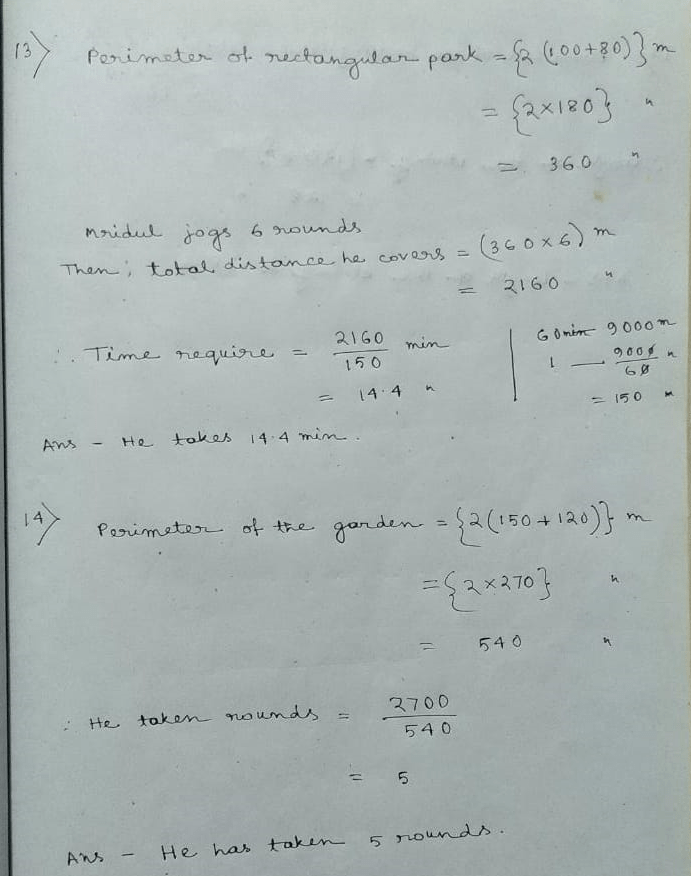# New Learning Composite Mathematics Class 6 SK Gupta Anubhuti Gangal Perimeter and Area Chapter 14A Solution

## New Learning Composite Mathematics Class 6 SK Gupta Anubhuti Gangal Perimeter and Area Chapter 14A Solution

(1) (a) Perimeter = (3 + 5 + 6 + 2 + 7) unit

= 23

(b) DE = (10 – 6) unit

= 4

EF = (8 + 7) unit

= 15

∴ Perimeter = (8 + 6 + 7 + 4 + 15 + 10) unit

= 50

(c) PQ = 3 Unit

ST = 2 Unit

TU = 3 Unit

WL = 2 Unit

∴ Perimeter = (1+2+3+1+3+2+1+2+3+1+3+2) Unit

= 24 Unit

(d) FG = 5 unit

∴ Perimeter = (5+0.5+3+3+0.5+5+1) Unit

= 18 Unit

(e) ∴ Perimeter = (1+3+2+3+4+1+3+2+3+4+1+3+2+3+4+1+3+2+3+4) Unit

= 52 Unit

(2) According to statement

(7 + a + 5 + 9 + 4 + 16) = 53

Or, a = 53 – (7 + 5 + 9 + 4 + 16)

Or, a = 53 – 41

∴ = 12

Ans – 12m

(3) (a) Perimeter = (3 X 8) cm

= 24 cm

(b) Perimeter = (4 X 10) cm

= 40 cm

(c) Perimeter = (5 X 62) cm

= 31

(d) Perimeter = (6 X 9.5) cm

= 57 cm

(e) Perimeter = {(2 X 5) + 7} cm

= {10 + 7} cm

= 17 cm

(4) (a) Length at one side = 36.8/4 cm

= 9.2 cm

(b) Length of one side = 22.5/3 cm

= 7.5 cm

(c) Length of one side = 42.4/8 cm

5.3

(d) Length of one side = 25.2/4 cm

= 6.3

(5) (a) Length of each side = 48/4 cm

= 12 cm

(b) Length of each side = 48/3 cm

= 16

(c) Length of each side = 48/6 cm

= 8 cm

(6) 2a + 14 = 50

Or, 2a = 50 – 14

Or, 2a = 36

Or, a = 36/2

∴ a = 18

Ans – 18 cm

(7) (a) Perimeter of the rectangle = {2 (8+5)} cm

= 2 X 13

= 26

(b) Perimeter of the rectangle = {2 (10.7 + 7.3)} cm

{2 X 18} cm

= 36

(c) 90 mm = 9cm

∴ Perimeter of the rectangle = {2 (12+9)} cm

= {2 X 21} cm

= 42 cm

(d) 0.85m = 85 cm

∴ Perimeter of the rectangle = {2 (85 + 70} cm

= {2 X 155} cm

= 310 cm

= 3.10 m

(8) (a) Let, length of the rectangle be L

2 (L + 5) = 32

Or, 2L + 10 = 32

Or, 2L = 32 – 10

Or, 2L = 22

Or, L = 22/2

∴ L = 11

Ans – Length of the rectangle is 11 cm

2 (x + 24) = 80

Or, 2x + 48 = 80

Or, 2x = 80 – 48

Or, 2x = 32

Or, x = 32/2

∴ x = 16

Ans – The breadth of the rectangle is 16 cm.

(9) Length of the rectangle = (15 X 3) cm

= 45

∴ Perimeter of the rectangle = (15 X 3) cm

= 45

∴ Perimeter = {2 (45 + 15)} cm

= 2 X 60 cm

= 120 cm

(10) Ratio of length and width = 5:3

Let, length = 5x

Width = 3x

2 (5x + 3x) = 112

Or, 2 X 8x = 112

Or, 8x = 112/2

Or, x = 112/2X8 = 7

∴ Length = (5 X 7) cm

= 35 cm

Width = (3 X 7) cm

= 21 cm

Ans – The length and the width of the rectangle is 35 cm, 21 cm.

(11) Perimeter of the square park = (4 X 80) cm

= 320 cm

Perimeter of the rectangle park = {2 X (65 + 55) cm

= {2 X 120} cm

= 240 cm

Ans – From runs greater distance.

(12) Perimeter = {2 (850 + 700)} m

= {2 X 1550} m

= 3100 cm

∴ Wires requires = (5 X 3100) m

= 15500 m

Then, cost of fencing = RS (15500 X 80)

= RS 1240000

Ans – length of the wire is 15500 m cost of fencing is RS 1240000.Updated: June 14, 2021 — 8:57 pm